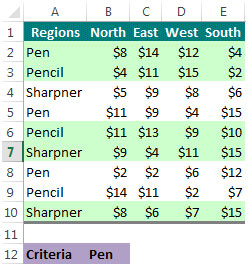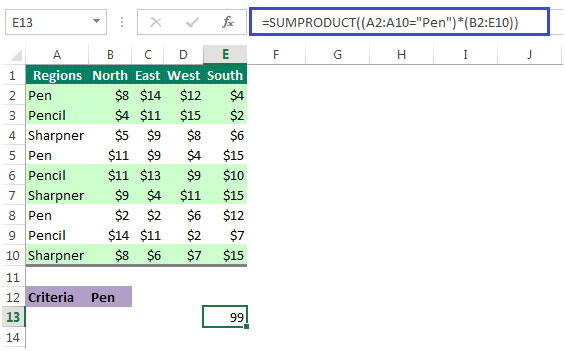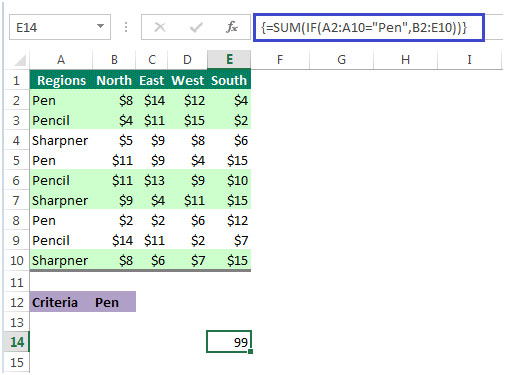# Sum Multiple columns based on Criteria in Microsoft Excel 2010

In Excel, with a specific criteria we can add the sum of multiple columns. We can create a formula using the Sum, If and Sumproduct functions.

Let’s take an example:

• Column A has Pen, Pencil, etc.
• Regions in columns B:E i.e. North, East, West, South as shown in the below picture.
• Here, we want to find the total value for Pen in all the regions• In cell E13 we want to show the total value of Pen in the range B2:E10
• To get the result in cell E13,the formula would be =SUMPRODUCT((A2:A10="Pen")*(B2:E10))
• This is what our result looks like -If you manually add up the values for columns B:E where column A contains “Pen”, you will see that it adds up to 99, which we have also derived from the formula.

Now lets understand the Sumproduct function.

SUMPRODUCT: SUMPRODUCT function multiplies the corresponding items in the arrays and returns the sum of the results.

#### Syntax =SUMPRODUCT(array1,array2,array3,...)

We can follow another method to do the same calculation. We can use Sum & IF together to get the desired result as follows:

Formula in cell E14 would be =SUM(IF(A2:A10="Pen",B2:E10))along with CTRL + SHIFT + ENTERThis is an array formula & has to be enclosed with CSE(Ctrl + Shift + Enter keys on the keyboard).

IF: Checks whether a condition is met, and returns one value if TRUE, and another value if FALSE

#### Parameters:

Logical test is the condition or a value that you want to test.
value_if_true is optional. It is the value that is returned if the condition is TRUE.
value_if_false is optional. It is the value that is return if the condition is FALSE.

Sum: Adds all the numbers in a range of cells

#### Syntax =SUM(number1,number2,...)

1.Karthikeyan Subbiyan

Wonderfull!
Thank you very much for this tip 🙂

2.Hi Linn

3.